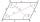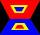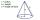# Rhombus angles

If one angle in the rhombus is 159°, what is it neighboring angle in rhombus?

Result

neighboring angle =  21 °

#### Solution:Leave us a comment of example and its solution (i.e. if it is still somewhat unclear...):

Showing 0 comments:Be the first to comment!## Next similar examples:

1. DiagonalCan a rhombus have the same length diagonal and side?
2. Angles 1It is true neighboring angles have not common arm?
3. ParallelogramIn the parallelogram we know one internal angle 67°33`. Calculate the other internal angles.
4. Rectangular trapezoidHow many inner right angles has a rectangular trapezoid?
5. Obtuse angleWhich obtuse angle is creating clocks at 17:00?
6. Dropped sheetsThree consecutive sheets dropped from the book. The sum of the numbers on the pages of the dropped sheets is 273. What number has the last page of the dropped sheets?
7. Find xSolve: if 2(x-1)=14, then x= (solve an equation with one unknown)
8. The diagramThe diagram is a cone of radius 8cm and height 10cm. The diameter of the base is. ..
9. RoundingThe following numbers round to the thousandth:
10. Pizza 4Marcus ate half pizza on monday night. He than ate one third of the remaining pizza on Tuesday. Which of the following expressions show how much pizza marcus ate in total?
11. Write 2Write 791 thousandths as fraction in expanded form.
12. HotelThe hotel has a p floors each floor has i rooms from which the third are single and the others are double. Represents the number of beds in hotel.
13. Passenger boatTwo-fifths of the passengers in the passenger boat were boys. 1/3 of them were girls and the rest were adult. If there were 60 passengers in the boat, how many more boys than adult were there?
14. Simple equationSolve the following simple equation: 2. (4x + 3) = 2-5. (1-x)
15. DisjointHow many elements have union and intersection of two disjoint sets when the first have 1 and secodn 8 elements.
16. Equation 29Solve next equation: 2 ( 2x + 3 ) = 8 ( 1 - x) -5 ( x -2 )
17. Unknown number 11That number increased by three equals three times itself?